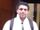## Find numeric values in character

Hi!

I'm trying to find only those variables, where first 2 symbols are letters and next 8 are numbers and length of variable is 10 digits. I used code: (anyalpha(substr(CODE, 1, 2), 2) and anydigit(substr(CODE, 3, 10), 😎 and length(CODE)=10). But it doesn't work. Please help to do it.

Have:

AB12345678

ABCD201642
20AB3413089000788913
20AB00000247287021

CD12345678

Want:

AB12345678

CD12345678

1 ACCEPTED SOLUTION

Accepted Solutionsnovinosrin
Tourmaline | Level 20

## Re: Find numeric values in character

``````
data have;
input var \$20.;
cards;
AB12345678
ABCD201642
20AB3413089000788913
20AB00000247287021
CD12345678
;

data want;
set have;
where lengthn(var)=10;
if prxmatch('/^[a-z]{2}\d{8}/i', var);
run;
``````
4 REPLIES 4novinosrin
Tourmaline | Level 20

## Re: Find numeric values in character

``````
data have;
input var \$20.;
cards;
AB12345678
ABCD201642
20AB3413089000788913
20AB00000247287021
CD12345678
;

data want;
set have;
where lengthn(var)=10;
if prxmatch('/^[a-z]{2}\d{8}/i', var);
run;
``````

## Re: Find numeric values in character

Thanks a lot, novinosrin!

## Re: Find numeric values in character

Let's try using the ANYDIGIT and ANYALPHA functions to get the information needed for your test.

First let's make some data.

``````data have ;
input code \$30. ;
cards;
AB12345678
ABCD201642
20AB3413089000788913
20AB00000247287021
CD12345678
XX1234 678
;``````

Now let's make some tests and then check that they follow all of the rules. Let's use NOTDIGIT to make sure the last 8 characters are all digits.

``````data want;
set have ;
len = lengthn(code);
first_digit= anydigit(code);
last_digit= anydigit(code,-len);
last_nondigit=notdigit(code,-len);
first_alpha=anyalpha(code);
last_alpha=anyalpha(code,-len);
want=len=10 and first_digit=3 and last_digit=10 and last_nondigit=2 and first_alpha=1 and last_alpha=2;
run;``````

Results:

```                                      first_    last_      last_     first_    last_
Obs    code                    len     digit    digit    nondigit     alpha    alpha    want

1     AB12345678               10       3        10         2          1        2        1
2     ABCD201642               10       5        10         4          1        4        0
3     20AB3413089000788913     20       1        20         4          3        4        0
4     20AB00000247287021       18       1        18         4          3        4        0
5     CD12345678               10       3        10         2          1        2        1
6     XX1234 678               10       3        10         7          1        2        0```

## Re: Find numeric values in character

Thanks, Tom. Your solution seems good too.
Discussion stats
• 4 replies
• 750 views
• 2 likes
• 3 in conversation